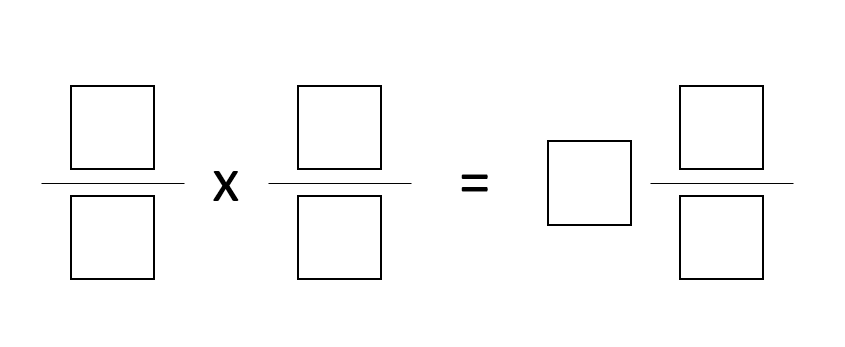# Multiplying Two Fractions to Get a Mixed Number

Directions: Using the digits 1 to 9, at most one time each, fill in the boxes to make a true statement.### Hint

How does choosing the whole number affect the digits you choose for the fractions you multiply together?

Source: Joseph Nguyen

## Volume of Rectangular Prisms 2

Directions: Using the digits 1 through 9, at most one time each, place a digit …

1.This really helped.

•2.Another answer is 8/6 * 4/3 = 1 7/9

3.One of my students found 7/4 * 8/6 = 2 1/3

4.5.6.7.how do you put in the numbers?

•You don’t you just do it on paper or in your head

8.Another one is 3/1 times 5/2 equals 7 1/2